# MARS-ROBOTICS PROGRAMMERS

### FTC TEAMS: 5187 - 11462 - 6206

- Established: MHS 2017 -
This is a programmers section aimed at teaching new programmers, having introductory resources, and guides on configuring robots.

## Installing & Configuring Android SDK

If the environment is already setup for you, continue on to the "Syntax" section.

Windows, MacOS, Linux

This button will take you to the Android SDK install guide.
Select which operating system you would like help with:
Windows, MacOS, Linux

This button will take you to FTC App Repository on GitHub.
The app is continually updated on GitHub, with instructions on how to download it.
How you manage your code, and whether or not you choose to use GitHub is up to you.

This button will take you to the FTC App Documentation on GitHub.
The Mars-Robotics programmers page is only a brief introduction.
The Official Documentation is very detailed, glance over it if you have further questions.

# The Basics of JAVA

### COMMON JAVA KEYWORDS

```"abstract" // an abstract class or method
"assert" // with assertions enabled, throws an error if condition not fulfilled
"boolean" // the Boolean type with values true and false
"break" // breaks out of a switch or loop
"byte" // the 8-bit integer type
"case" // a case of a switch
"catch" // the clause of a try block catching an exception
"char" // the Unicode character type
"class" // defines a class type
"comments" // A feature that allows documentation to be ignored by the compiler. (// or /* ABC */)
"continue" // continues at the end of a loop
"default" // 1) the default clause of a switch, 2) denotes default implementation of an interface method
"do" // the top of a do/while loop
"double" // the double-precision floating-number type, Double is more complex than int & for bigger numbers
"else" // the else clause of an if statement
"enum" // an enumerated type
"extends" // defines the parent class of a class
"final" // a constant, or a class or method that cannot be overridden
"finally" // the part of a try block that is always executed
"float" // the single-precision floating-point type, basially for decimals
"for" // a loop type
"if" // a conditional statement
"implements" // defines the interface(s) that a class implements
"import" // imports a package
"instanceof" // tests if an object is an instance of a class
"int" // the 32-bit integer type
"interface" // an abstract type with methods that a class can implement
"long" // the 64-bit long integer type
"main()" // Is the default method, usually in the format of "public static void main()"
"native" // a method implemented by the host system
"new" // allocates a new object or array
"null" // a null reference
"package" // a package of classes
"private" // a feature that is accessible only by methods of this class
"protected" // a feature that is accessible only by methods of this class, its children, and other classes in the same package
"public" // a feature that is accessible by methods of all classes
"return" // returns from a method
"short" // the 16-bit integer type
"static" // a feature that is unique to its class, not to objects of its class
"strictfp" // Use strict rules for floating-point computations
"string" // The use of multiple characters, Ex: "Hello"
"super" // invoke a superclass constructor or method
"switch" // a selection statement
"synchronized" // a method or code block that is atomic to a thread
"this" // the implicit argument of a method, or a constructor of this class
"throw" // throws an exception
"throws" // the exceptions that a method can throw
"transient" // marks data that should not be persistent
"try" // a block of code that traps exceptions
"void" // denotes a method that returns no value
"volatile" // ensures that a field is coherently accessed by multiple threads
"while" // a loop
```

### VARIABLES, OPERATORS, & MATH

```// VARIABLES
int num = 1; // Variable "num" is equal to integer "1"
char letter = 'A'; // Variable "letter" is equal to 'A'
String str = "Hello World"; // Variable "str" is equal to "Hello World"
float dec = 1024.45; // Variable "dec" is equal to the decimal "1024.45"
double bigint = 99999999; // Variable "bigint" is equal to "999999999"
int array[] = { 5, 10, 15, 20, 25, 30, 35, 40 }; // An array can hold several objects, in this case intergers

// OPERATORS
if (num == 1) {code} // If var num is equal(==) to 1, runs {code}
if (num != 1) {code} // If var num is not(!) equal(=) to 1, runs {code}
if (num > 0) {code} // If var num is greater(>) than 0, runs {code}
if (num < 2) {code} // If var num is less(<) than 2, runs {code}
if (num >= 1) {code} // If var num is greater(>) than or equal(=) to 1, runs {code}
if (num <= 1) {code} // If var num is less(<) than or equal(=) to 1, runs {code}
if (num > 0 && num < 10) {code} // If var num is between 0 AND(&&) 10, runs {code}
if (num > 0 || num < 10) {code} // If var num is greater than 0, OR(||) less than 10 runs {code}
if (!(num > 0 && num < 10)) {code} // If var num is not between 0 and 10, runs {code}
if (num % 2) {code} // If var num has remainder(%) of 2, runs {code}

// MATH -- Add, Subtract, Multiply, & Divide (Example: num = 1)
int foo = num + num; // Var "foo" is equal to integer of var "num" + "num",
int foo = ( 2 = 1 + 1 ) // Example

int foo = num - num; // Var "foo" is equal to integer of var "num" - "num",
int foo = ( 0 = 1 - 1 ) // Example

int foo = num * num; // Var "foo" is equal to integer of var "num" * "num",
int foo = ( 1 = 1 x 1 ) // Example

int foo = num / num; // Var "foo" is equal to integer of var "num" / "num",
int foo = ( 1 = 1 / 1 ) // Example

// Math Operations
num++; // Var num is increased by 1
num--; // Var num is decreased by 1

num == 2 // Example of var num is already equal to 2
num += 10; // var num is equal to 2 + 10; num = 12
num -= 10; // var num is equal to 2 - 10; num = 8
num *= 10; // var num is equal to 2 x 10; num = 20
num /= 2; // Var num is equal to 2 / 2; num = 1
```

### Basic Java Class Structure

```// This tells the app that this is our opmode
package org.firstinspires.ftc.teamcode;

// An import allows you to add classes and functionality from other packages
// This specific import adds opmode support
import com.qualcomm.robotcore.eventloop.opmode.OpMode;

// This specific import adds dc motor hardware support
import com.qualcomm.robotcore.hardware.DcMotor;

// This tells the Driver Phone where to put the opmode and what to call it
@TeleOp(name="TeamName", group="Example")

// A class is a starting container for your code, this class extends another class: OpMode
public class ExampleTest extends OpMode { // Class Opening Curly Bracket

//This is the area which you should declare your variables for the program.
// A private variable means it can only be used within this program.
// Null means it is equal to nothing.
private DcMotor leftMotor1 = null;

// An @Override Changes the scope of this method to only this program.
@Override
public void init() { // Init Opening Curly Bracket

// Place your init code here
// The init() method runs once, which is mainly used for hardware mapping.

} // Init Closing Curly Bracket

@Override
public void init_loop() { // InitLoop Opening Curly Bracket

// Place your init_loop code here
// The init_loop() method runs in a loop during the init which is primarily used for real-time telemetry.

} // InitLoop Closing Curly Bracket

@Override
public void start() { // Start Opening Curly Bracket

// Place any code here that needs to start at the same time as the loop, ex: time
// The start() method to run once when the driver hits play.

} // Start Closing Curly Bracket

@Override
public void loop() { // Loop Opening Curly Bracket

// Place your Main Loop code here
// Code to run repeatedlty (LOOPS) after the driver hits play but before they hit stop
// This is the main loop where you enter all your loob methods to enable them. This loop is the actual running program.

} // Loop Closing Curly Bracket

@Override
public void stop() { // Stop Opening Curly Bracket

// Place any code here that needs to run when the player hits stop.
// Example: telemetry.

} // Stop Closing Curly Bracket

} // Class Closing Curly Bracket

```

Just a simple java class that shows how java code should be formatted, the basic methods used for the first app, and how they are executed operatively. Knowing which method to place your code in makes a large programming task much easier. Sticking to the proper format and commenting your code makes your code easier to read for others.

# ANDROID PHONES

### Phone Setup & Building

The entire phone setup & configuration process is very long & informative, which can be found here:

### Building from Android SDK

Transferring the code from Android SDK to the Robot Controller requires you to build.

#### Step 1: Build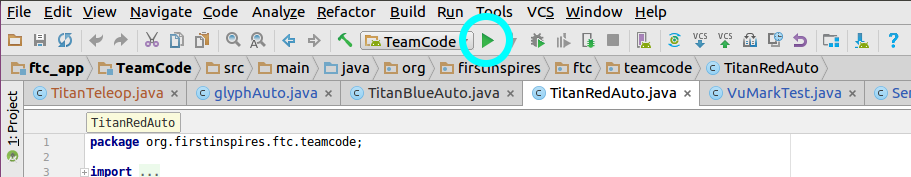The cyan circle shows the location of the run button. Press the run button when you would like to build a class to the phone.

#### Step 2: Select Device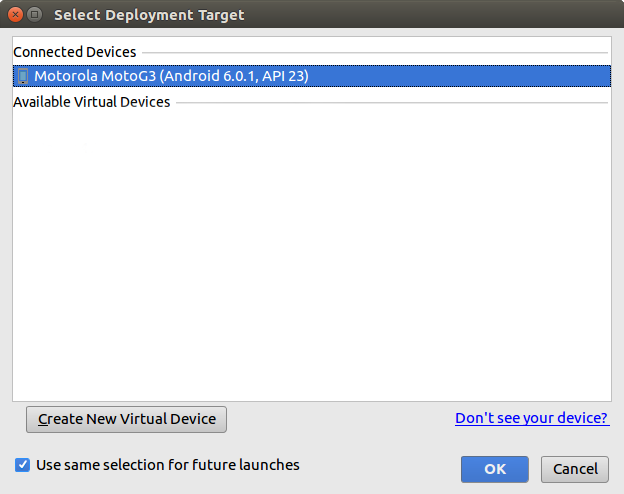After pressing the run button, in the popup asking you to select your android device, select your phone and click "OK".

#### Step 3: Build Runs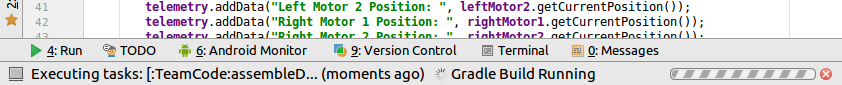At the bottom of the editor, the bottom bar will show you the build process as its running.

#### Step 4: Build Finishes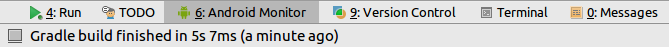When the build is finished, it will state that it is finished along with how long it took.

#### Step 5: Restart App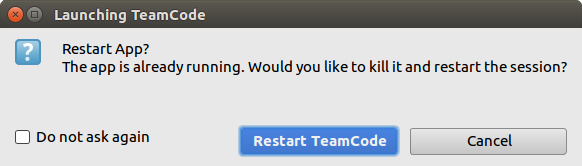When you connected the phone, if you have the robot app open while building, another popup will ask you to "Restart TeamCode".
The app will then proceed to restart on your android device.

## SAMPLE CODE

#### FOR REFERENCE AND TEACHING NEW PROGRAMMERS.

This button will take you to the sample code page.